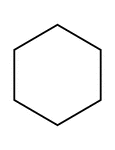### Flashcard of a polygon with six equal sides

A flashcard featuring an illustration of a polygon with six equal sides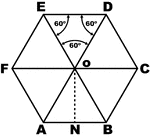### Regular Hexagon

Regular hexagon with equilateral triangles divided in the interior.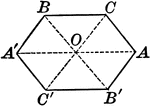### Axis of Symmetry Drawn on a Hexagon

Illustration of an axis of symmetry drawn with respect to a hexagon.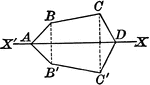### Axis of Symmetry Drawn on a Hexagon

Illustration of an axis of symmetry drawn with respect to a hexagon.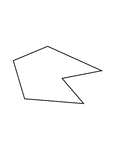### Irregular Concave Hexagon

Illustration of an irregular hexagon. This is also an example of a concave polygon.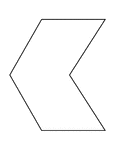### Irregular Concave Hexagon

Illustration of an irregular hexagon. This is also an example of a concave polygon with symmetry.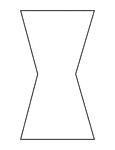### Irregular Concave Hexagon

Illustration of an irregular hexagon. This is also an example of a concave polygon with symmetry.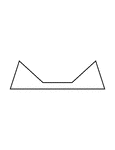### Irregular Concave Hexagon

Illustration of an irregular hexagon. This is also an example of a concave polygon with symmetry.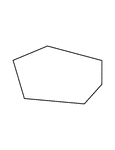### Irregular Convex Hexagon

Illustration of an irregular convex hexagon.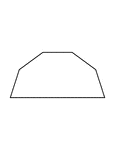### Irregular Convex Hexagon

Illustration of an irregular convex hexagon with symmetry.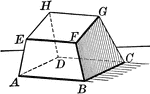### Hexahedron

Hexahedron - polyhedron with 6 faces.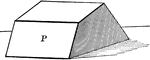### Hexahedron

Hexahedron - polyhedron with 6 faces.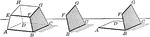### Hexahedron With Faces and Vertices

Hexahedron with faces and vertices shown.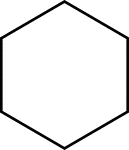### 6-sided Polygon

Polygon consisting of 6 sides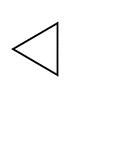### Fractions of 6-sided Polygon

1/6 of a 6 sided polygon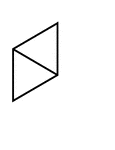### Fractions of 6-sided Polygon

2/6 of a 6 sided polygon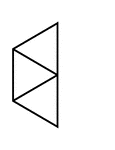### Fractions of 6-sided Polygon

3/6 of a 6 sided polygon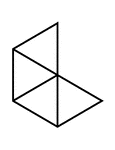### Fractions of 6-sided Polygon

4/6 of a 6 sided polygon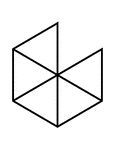### Fractions of 6-sided Polygon

5/6 of a 6 sided polygon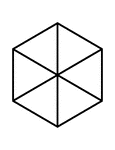### Fractions of 6-sided Polygon

6/6 of a 6 sided polygon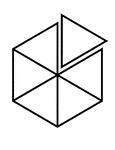### Fractions of 6-sided Polygon

6/6 of a 6 sided polygon with one piece detached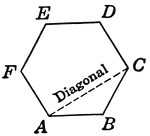### Hexagon Polygon

Hexagon with diagonal drawn.